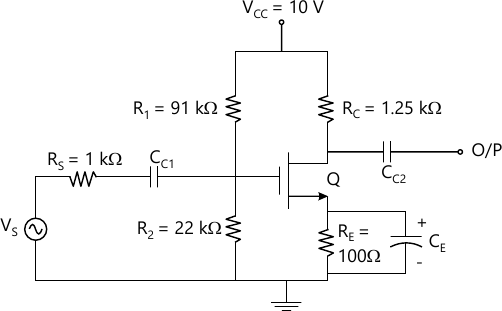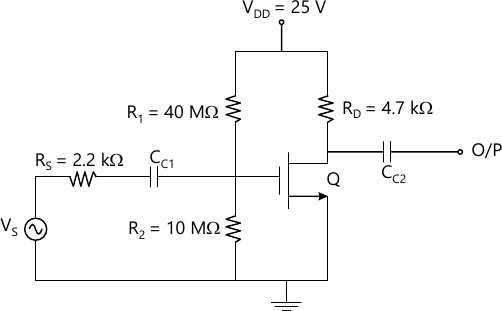MORE IN Electronic Devices & Circuits
SPPU Electronics and Telecom Engineering (Semester 3)
Electronic Devices & Circuits
December 2014
Total marks: --
Total time: --
INSTRUCTIONS
(1) Assume appropriate data and state your reasons
(2) Marks are given to the right of every question
(3) Draw neat diagrams wherever necessary

Answer any one question from Q1 and Q2
1 (a) Draw h-parameter models for CE, CB and CC transistor configurations.
6 M
1 (b) For the circuit shown in Fig 1. Silicon transistor with β=100 is used. Calculate IB, ,IC and VCE.6 M

2 (a) For the circuit shown in Fig 1. Silicon transistor with hie=1.1 KΩ, hfe=50, hre=2.5×10-4, hoe=25 μA/V is used. Calculate Ai, Av, Ri, Ri', R0 and R0'.
6 M

Answer any one question from Q3 and Q4
3 (a) Draw and explain Hartley oscillator using BJT. Calculate frequency of oscillation, when C=0.001 μF, L1=L2=100 μH and mutual inductance between L1 and L2 is 20 μH.
6 M
3 (b) Derive the expression for lower cut-off frequency and higher cut-off frequency in terms of percentage tilt and rise time respectively.
6 M

4 (a) Determine the input resistance of a series input connection and the output resistance of a shunt output connection for an ideal feedback voltage amplifier in which Av=105 and Aof=50. Assume that input and output resistances of the basic amplifier are Ri=10 KΩ and R0=20 KΩ respectively.
6 M
4 (b) Explain the effect of internal capacitance of transistor used in amplifier circuit on bandwidth of amplifier with frequency response curve. Define Fα and Fβ separately.
6 M

Answer any one question from Q5 and Q6
5 (a) Draw class B push pull power amplifier and show that Maximum efficiency is 78.5%.
6 M
5 (b) A transformer coupled class A power amplifier draws a current of 200 mA from a collector supply of 10 V when no signal is applied to it. Determine:
i) Maximum output power
ii) Maximum collector efficiency iii) Power rating of the transistors. If the load connected across transformer. Secondary is of 2Ω and transformer turns ratio is 5:1.
7 M

6 (a) Explain with circuit diagram, how even harmonics are eliminated in class B push-pull power amplifier.
6 M
6 (b) A sinusoidal signal VS=1.75 sin (600 t) is fed to a power amplifier. The resulting output current is I0=15 sin 600 t + 1.5 sin 1200 t+1.2 ssin 1800 t + 0.5 sin 2400 t. Calculate the percentage increase in the power due to distortion.
7 M

Answer any one question from Q7 and Q8
7 (a) Explain various non-ideal current voltage characteristics of EMOSFET.
6 M
7 (b) For the circuit shown in Fig 2. the MOSFET parameters are VT=3V, k=0.4 mA/V2. Determine VGS, VDS and ID and show that MOSFET is biased in the saturation region:7 M

8 (a) For the circuit shown in the Fig 2, determine gm, AD, Ri, Ri', R0 and R0'. Given VT=3V, k=0.4 mA/V2 and r0=40 KΩ.
7 M
8 (b) Describe Bi-CMOS technology of MOSFET with circuit diagram.
6 M

More question papers from Electronic Devices & Circuits